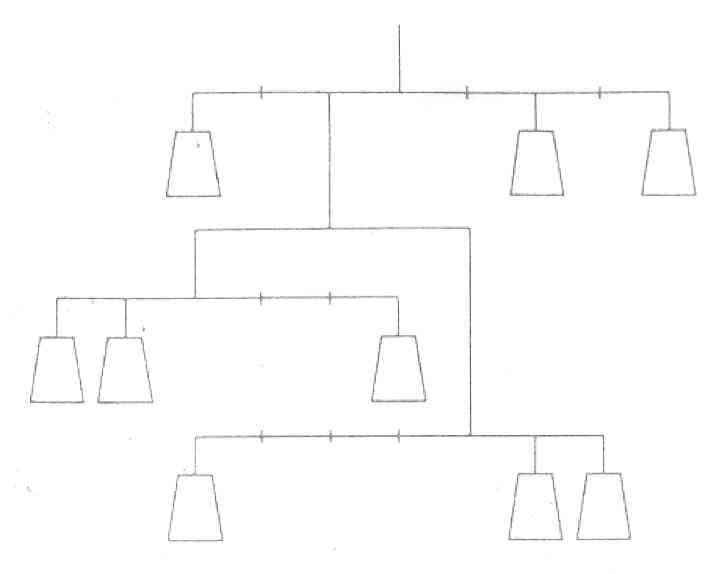# Individual Research Projects Section 6.4

### Project 6.2

Write out a derivation of the quadratic formula.

References:
You can check almost any high school algebra book.

### Project 6.3

Find any replacements for x, y, and z such that xn + yn  = zn, where n is greater than 2 and where x, y, and z are counting numbers. Write a history of this problem, known as Fermat’s Last Theorem.

References:
A source I recommend highly is the movie The Proof one of PBS’s shows on the NOVA series.

The web page for this move is:
http://www.pbs.org/wgbh/nova/proof/
Another general overview is at this site:
http://www-history.mcs.st-and.ac.uk/history/HistTopics/Fermat’s_last_theorem.html

### Project 6.4

Solve the following equation for x:

10 − 9(9 − 8(8 − 7(7 − 6(6 − 5(5 − 4(4 − 3(3 − 2(2 − x)))))))) = x

### Project 6.5

The idea of solving an equation sometimes uses the idea of a “balance” scale. If the balance point is not in the center, then the distance times the weight must be the same for the mobile to balance. For example, a weight of 2 units hanging three units from the pivot point will balance a 1 unit hanging 6 units from the pivot point. For the following puzzle, insert the weights through so that the mobile balances.